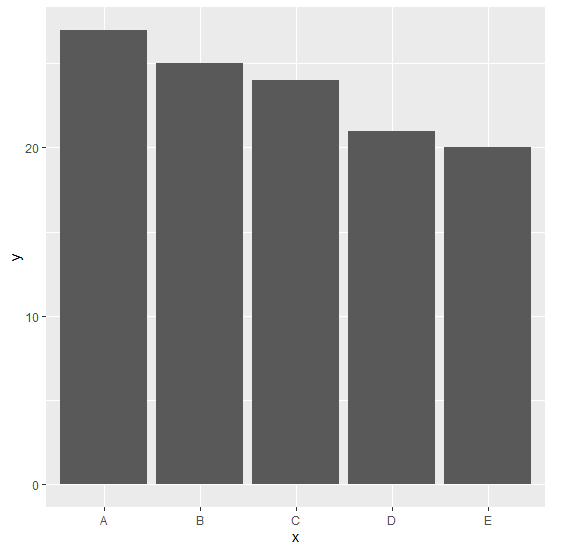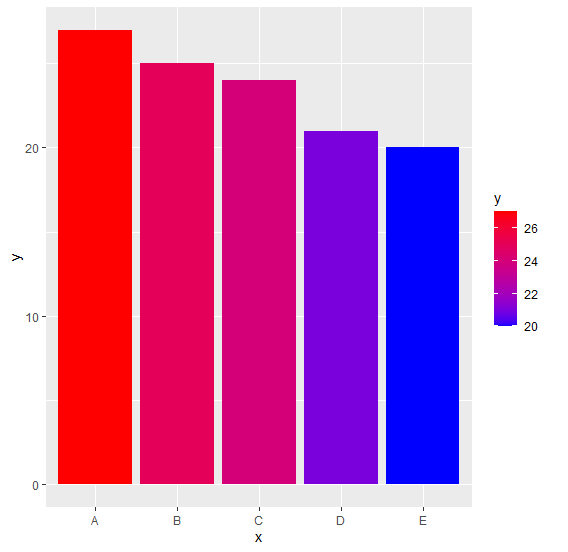# How to create bar plot with gradient colors using ggplot2 in R?n

To create bar plot with gradient colors using ggplot2, we can use scale_fill_gradient function where we can set the lower and higher color values.

For Example, if we have a data frame called df that contains two columns say Cat and Count then we can create the bar plot with gradient colors by using the below command −

ggplot(df,aes(Cat,Count,fill=Cat))+geom_bar(stat="identity")+scale_fill_gradient(low="blue",high="red")

## Example

Following snippet creates a sample data frame −

x<-LETTERS[1:5]
y<-c(27,25,24,21,20)
df<-data.frame(x,y)
df

The following dataframe is created

  x  y
1 A 27
2 B 25
3 C 24
4 D 21
5 E 20

To load ggplot2 package and create bar plot for data in df on the above created data frame, add the following code to the above snippet −

x<-LETTERS[1:5]
y<-c(27,25,24,21,20)
df<-data.frame(x,y)
library(ggplot2)
ggplot(df,aes(x,y))+geom_bar(stat="identity")

## Output

If you execute all the above given snippets as a single program, it generates the following Output −To create bar plot for data in df having bars filled with gradient color on the above created data frame, add the following code to the above snippet −

x<-LETTERS[1:5]
y<-c(27,25,24,21,20)
df<-data.frame(x,y)
library(ggplot2)
ggplot(df,aes(x,y,fill=y))+geom_bar(stat="identity")+scale_fill_gradient(low="blue",high="red")

## Output

If you execute all the above given snippets as a single program, it generates the following Output −